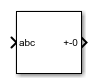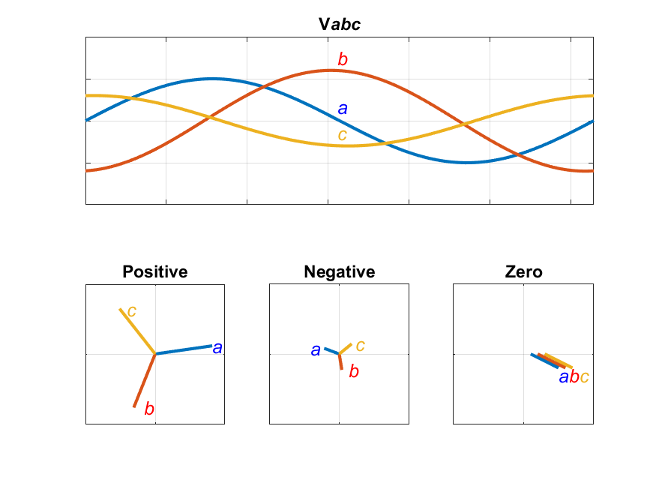# Symmetrical-Components Transform

Implement abc to +-0 transform

•Libraries:
Simscape / Electrical / Control / Mathematical Transforms

## Description

The Symmetrical-Components Transform block implements a symmetrical transform of a set of phasors. The transform splits an unbalanced set of three phasors into three balanced sets of phasors.

In an unbalanced system with balanced impedances, use this block to decouple the system into three independent networks. In a balanced system, use this block to simplify the set of three-phasors to an equivalent one-line network. In this case, the positive set represents the one-line network.

Use the `Power invariant` property to choose between the Fortescue transform, and the alternative, power-invariant version.

### Equations

The symmetrical-components transform separates an unbalanced three-phase signal given in phasor quantities into three balanced sets of phasors:

`$\left[\begin{array}{c}{v}_{a}\\ {v}_{b}\\ {v}_{c}\end{array}\right]=\left[\begin{array}{c}{v}_{a+}\\ {v}_{b+}\\ {v}_{c+}\end{array}\right]+\left[\begin{array}{c}{v}_{a-}\\ {v}_{b-}\\ {v}_{c-}\end{array}\right]+\left[\begin{array}{c}{v}_{a0}\\ {v}_{b0}\\ {v}_{c0}\end{array}\right],$`

where:

• va, vb, and vc make up the original, unbalanced set of phasors.

• va+, vb+, and vc+ make up the balanced, positive set of phasors.

• va-, vb-, and vc- make up the balanced, negative set of phasors.

• va0, vb0, and vc0 make up the balanced, zero set of phasors.

The block calculates the symmetric a-phase using the transformation:

`$\left[\begin{array}{c}{V}_{a+}\\ {V}_{a-}\\ {V}_{a0}\end{array}\right]=\frac{K}{3}\left[\begin{array}{ccc}1& a& {a}^{2}\\ 1& {a}^{2}& a\\ 1& 1& 1\end{array}\right]\left[\begin{array}{c}{V}_{a}\\ {V}_{b}\\ {V}_{c}\end{array}\right].$`

where a is the complex rotation operator

`$a={e}^{2\pi i/3},$`

and K is the constant that determines the type of transform:

To select the power-invariant transform and simplify the power calculation in the +-0 domain, enable the ```Power invariant``` property.

Because the remaining two sets of symmetrical phasors are not often used in calculation, the block does not calculate them. However, they are given in terms of simple rotations of the first set:

`$\left[\begin{array}{c}{V}_{b+}\\ {V}_{b-}\\ {V}_{b0}\end{array}\right]=\left[\begin{array}{ccc}{a}^{2}& 0& 0\\ 0& a& 0\\ 0& 0& 1\end{array}\right]\left[\begin{array}{c}{V}_{a+}\\ {V}_{a-}\\ {V}_{a0}\end{array}\right],$`

and

`$\left[\begin{array}{c}{V}_{c+}\\ {V}_{c-}\\ {V}_{c0}\end{array}\right]=\left[\begin{array}{ccc}a& 0& 0\\ 0& {a}^{2}& 0\\ 0& 0& 1\end{array}\right]\left[\begin{array}{c}{V}_{a+}\\ {V}_{a-}\\ {V}_{a0}\end{array}\right].$`

### Operating Principle

The three sets of balanced phasors generated by the transform have the following properties:

• The positive set has the same order as the unbalanced set of phasors a-b-c.

• The negative set has the opposite order as the unbalanced set of phasors a-c-b.

• The zero set has no order because all three phasor angles are equal.

This diagram visualizes the separation performed by the transform.In the diagram, the top axis shows an unbalanced three-phase signal with components a, b, and c. The bottom set of axes separates the three-phase signal into symmetrical positive, negative, and zero phasors.

Observe that in each case, the a, b, and c components are symmetrical and are separated by:

• +120 degrees for the positive set.

• -120 degrees for the negative set.

• 0 degrees for the zero set.

### Inverse Transform

The symmetrical-components transform is unique and invertible:

`$\left[\begin{array}{c}{V}_{a}\\ {V}_{b}\\ {V}_{c}\end{array}\right]=\frac{1}{K}\left[\begin{array}{ccc}1& 1& 1\\ {a}^{2}& a& 1\\ a& {a}^{2}& 1\end{array}\right]\left[\begin{array}{c}{V}_{a+}\\ {V}_{a-}\\ {V}_{a0}\end{array}\right].$`

Use the Inverse Symmetrical-Components Transform block to perform this inverse transform.

## Ports

### Input

expand all

Three-phase set of unbalanced phasors to be separated, given as a complex signal.

Data Types: `single` | `double`

### Output

expand all

Positive, negative and zero a phasors, output as a complex signal. Use the rotations given in the equations section to compute the b and c phasor sets.

Data Types: `single` | `double`

## Parameters

expand all

Power invariant toggle. Select this parameter to use the power-invariant alternative of the original Fortescue transform.

 Anderson, P. M. Analysis of Faulted Power Systems. Hoboken, NJ: Wiley-IEEE Press, 1995.